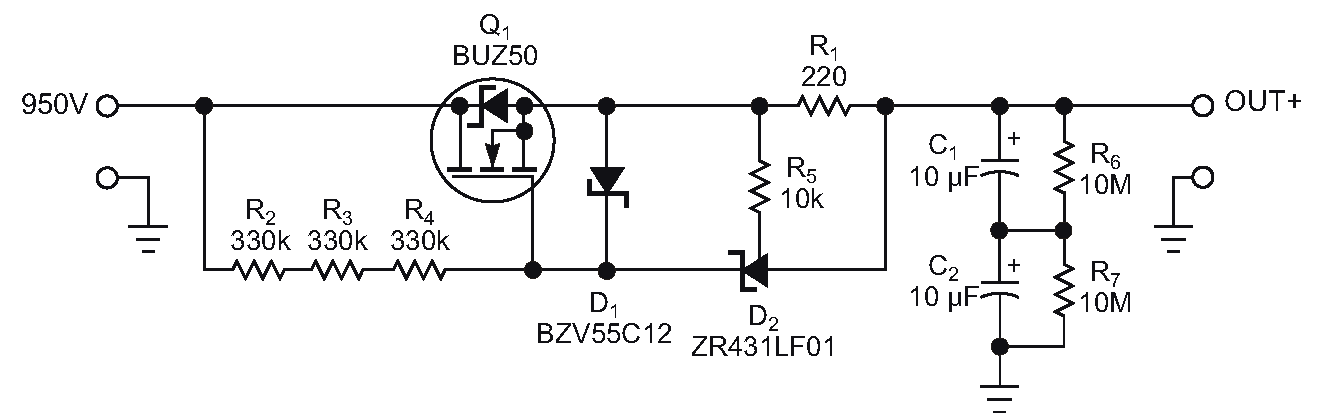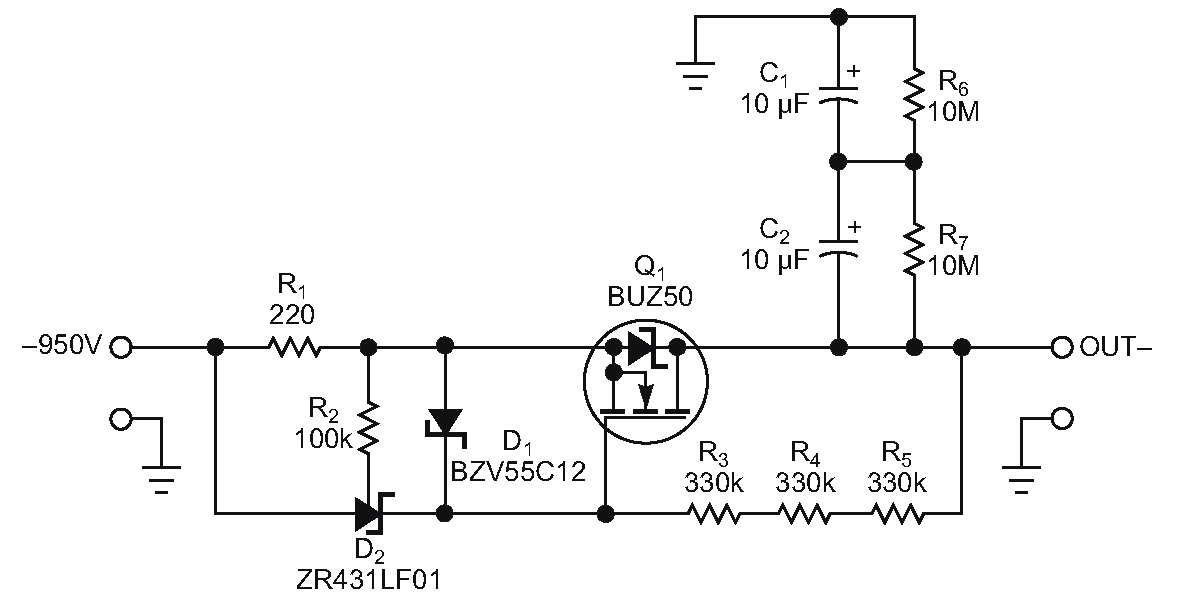# Produce current from positive or negative high-voltage supplies

## ZR431LF01TA

EDN

You sometimes need a current source for supply voltages as high as 1000 V or more. This current source can be useful for ripple-voltage reduction when the current source’s high-impedance node feeds an electrolytic capacitor to effectively short the ripple voltage. The circuit in Figure 1 does the job with a temperature-stable and exact-output current. The circuit uses N-channel MOSFET Q1, which has a drain-to-source voltage of 1000 V. Zener diode D2, a ZR431LF01 shunt regulator, stabilizes and regulates the output current. The threshold voltage of Q1 must be higher than D2’s 1.25 V reference voltage.Figure 1. This circuit produces current using positive voltages.

R1 and the voltage across it determine the output current. In this case, 1.25 V/220 Ω = 5.6 mA. D2 regulates the MOSFET’s gate-to-source voltage until the voltage across R1 equals D2’s reference voltage, which is temperature stable and accurate, making the current source stable and accurate, as well. Zener diode D1 protects Q1’s gate and limits the gate-to-source voltage if no load connects to the current source.Figure 2. This modified version of the circuit in Figure 1 produces current using negative voltages.

You can use a similar circuit to get constant current from negative high-voltage supplies even if a P-channel MOSFET with a high drain-to-source voltage is unavailable. To make the circuit work with negative supplies with an N-channel MOSFET, you must modify the circuit in Figure 1. You can use the N-channel MOSFET by changing the source and drain connections of MOSFET Q1 in Figure 2. The function of the current regulation with zener diode D2 is the same as for positive voltages.

EDN